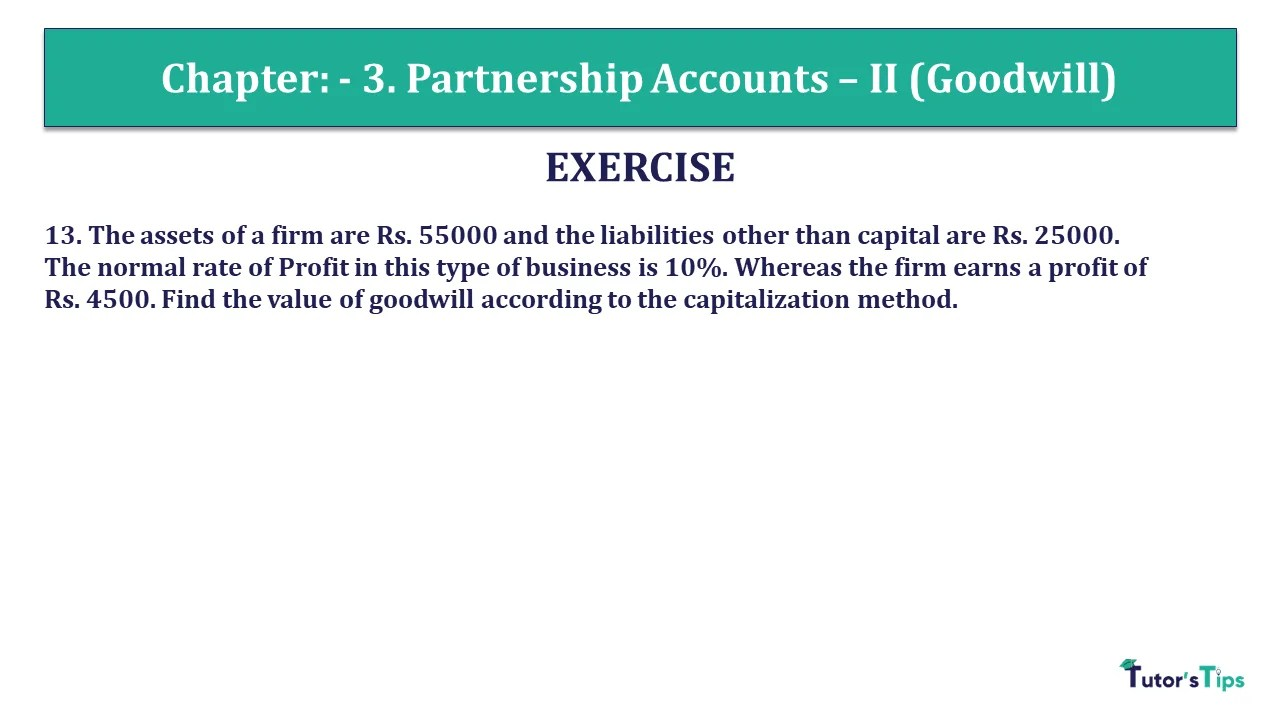# Question 13 Chapter 3 – Unimax Class 12 Part 1 – 2021Question 13 Chapter 3 – Unimax Class 12 Part 1

13. The assets of a firm are Rs. 55000 and the liabilities other than capital are Rs. 25000. The normal rate of Profit in this type of business is 10%. Whereas the firm earns a profit of Rs. 4500. Find the value of goodwill according to the capitalization method.

## The solution of Question 13 Chapter 3 – Unimax Class 12 Part 1:

Capital Employed = Total Assets – Outside habities
= Rs. 55000 – Rs. 25000
= Rs. 30000
Capitalised value of average profits (i.e. Rs. 4500) at 10%
Average Profits/Normal rate of return
Rs. 4500/10/100Or Rs. 4500 X 100/10 = Rs. 45000
Goodwill = Capitalized average profits – Actual Capital employed
= Rs. 45000 – Rs. 30000
= Rs. 15000

What is Partnership – Meaning and its Types

T.S. Grewal’s Double Entry Book Keeping +2 (Vol. I: Accounting for Not-for-Profit Organizations and Partnership Firms)